Next: Hooke's law Up: Conservation of energy Previous: Conservative and non-conservative force-fields

## Potential energy

Consider a body moving in a conservative force-field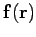. Let us arbitrarily pick some pointin this field. We can define a function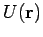which possesses a unique value at every point in the field. The value of this function associated with some general pointis simply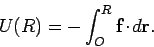(155)

In other words,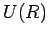is just the energy transferred to the field (i.e., minus the work done by the field) when the body moves from pointto point. Of course, the value of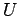at pointis zero: i.e.,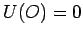. Note that the above definition uniquely specifies, since the work done when a body moves between two points in a conservative force-field is independent of the path taken between these points. Furthermore, the above definition would make no sense in a non-conservative field, since the work done when a body moves between two points in such a field is dependent on the chosen path: hence,would have an infinite number of different values corresponding to the infinite number of different paths the body could take between pointsand.

According to the work-energy theorem,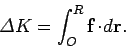(156)

In other words, the net change in the kinetic energy of the body, as it moves from pointto point, is equal to the work done on the body by the force-field during this process. However, comparing with Eq. (155), we can see that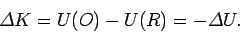(157)

In other words, the increase in the kinetic energy of the body, as it moves from pointto point, is equal to the decrease in the functionevaluated between these same two points. Another way of putting this is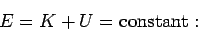(158)

i.e., the sum of the kinetic energy and the functionremains constant as the body moves around in the force-field. It should be clear, by now, that the functionrepresents some form of potential energy.

The above discussion leads to the following important conclusions. Firstly, it should be possible to associate a potential energy (i.e., an energy a body possesses by virtue of its position) with any conservative force-field. Secondly, any force-field for which we can define a potential energy must necessarily be conservative. For instance, the existence of gravitational potential energy is proof that gravitational fields are conservative. Thirdly, the concept of potential energy is meaningless in a non-conservative force-field (since the potential energy at a given point cannot be uniquely defined). Fourthly, potential energy is only defined to within an arbitrary additive constant. In other words, the point in space at which we set the potential energy to zero can be chosen at will. This implies that only differences in potential energies between different points in space have any physical significance. For instance, we have seen that the definition of gravitational potential energy is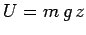, whererepresents height above the ground. However, we could just as well write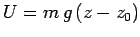, where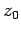is the height of some arbitrarily chosen reference point (e.g., the top of Mount Everest, or the bottom of the Dead Sea). Fifthly, the difference in potential energy between two points represents the net energy transferred to the associated force-field when a body moves between these two points. In other words, potential energy is not, strictly speaking, a property of the body--instead, it is a property of the force-field within which the body moves.Next: Hooke's law Up: Conservation of energy Previous: Conservative and non-conservative force-fields
Richard Fitzpatrick 2006-02-02